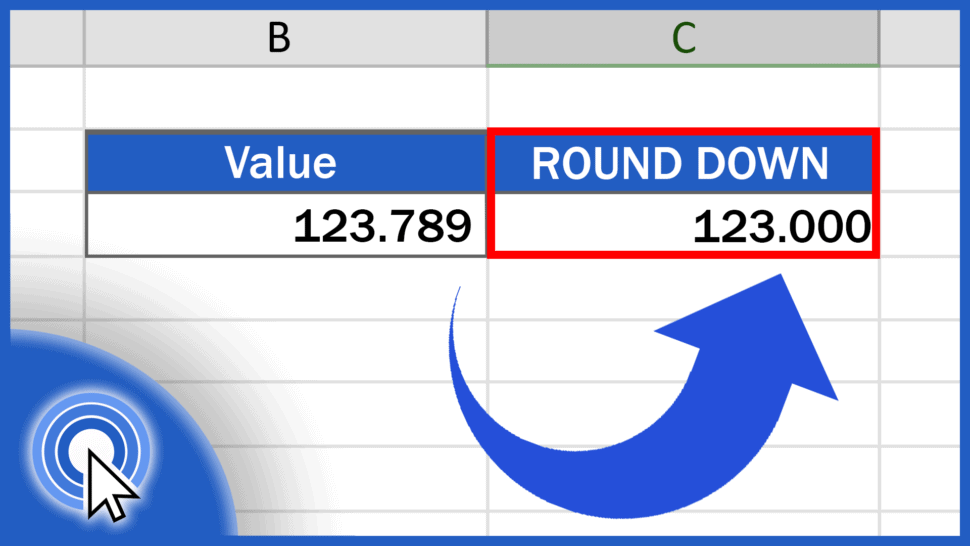# How to ROUND DOWN in ExcelIn today’s tutorial, we’re going to talk about numbers, specifically how to round down in Excel.

Thanks to the ROUND DOWN function, Excel will always round values down, based on the given number of decimal places.

Together, we’ll have a look at how to use this function effectively. Let’s start now!

See the video tutorial and transcription below:

Contents

As we already know, Excel offers multiple functions when it comes to calculations of any kind.

The same goes for rounding numbers. We can round up, round down, or simply round numbers according to the general mathematical rule.

Each function will help you round numbers in a different way and each is going to be presented in a separate tutorial. You’ll find the links to these videos below.

In this tutorial, we’re gonna have a closer look at the function ROUND DOWN.

Let’s say we want to round down the value in the column B in different ways. We’ll take a look at how to work with various numbers of decimal places, or what to do if we want to make the result a whole number.

## How to ROUND DOWN to one decimal place in Excel

Click into the cell C3, enter the equal sign and type in the function ‘ROUNDDOWN’. Then carry on with an opening bracket and here we’ll need to include two things.

The first one will be the cell which contains the number we want to round down, so click on B3, then enter a comma and you can type in the second value – the number which identifies how many decimal places there should be in the result.

So, if you want to round down to one decimal place, type ‘1’, close the parentheses, and hit ‘Enter’. In C3, Excel will show the result – the number from B3 rounded down to one decimal place.

## How to ROUND DOWN to two decimal places in Excel

If you want to round down to two decimal places, simply use 2 in the function and the resulting number will be rounded down to two decimal places.

By using positive numbers like 1, 2, 3, and so on, you can round down to any number of decimal places.

But let’s move on, now.

## How to round down the value up to make it a whole number

To round the value down to make it a whole number, enter a zero in the function,

and Excel will do just what you want – it will round the original value down making it a whole number, like this.

And we’re not finished yet!

## How to round down a number up to tens or hundreds

To round a number down to tens or hundreds, which means moving to the left from the decimal point, you need to use negative numbers in the formula, so make sure you include the minus sign with the number in parentheses.

Here are a couple of examples.

To round the number down to the nearest ten, enter ‘-1’ into the formula and here comes the result!

If you want to round the number down to the nearest hundred, type in ‘-2’ and Excel will calculate it for you immediately.

And that’s how it works! You can use this function to round down any number just as you need.

If you’d like to know more about similar functions, like the function Round or Round Up, you can learn how to use them effectively by watching the rest of the video tutorials on rounding numbers by EasyClick Academy. Check out the links in the description below!

If you found this tutorial helpful, give us a like and watch other video tutorials by EasyClick Academy. Learn how to use Excel in a quick and easy way!

Is this your first time on EasyClick? We’ll be more than happy to welcome you in our online community. Hit that Subscribe button and join the EasyClickers!

Thanks for watching and I’ll see you in the next tutorial!